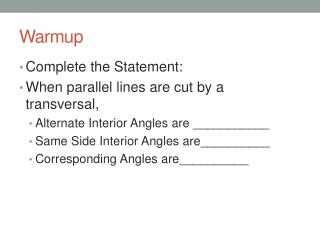DownloadDownload PresentationWarmup

# Warmup

Download Presentation## Warmup

- - - - - - - - - - - - - - - - - - - - - - - - - - - E N D - - - - - - - - - - - - - - - - - - - - - - - - - - -
##### Presentation Transcript

1. Warmup • Complete the Statement: • When parallel lines are cut by a transversal, • Alternate Interior Angles are ___________ • Same Side Interior Angles are__________ • Corresponding Angles are__________

2. 4.1 Congruent Figures

3. Learning Target • I can identify the corresponding parts of congruent figures

4. Congruent Polygons – Have congruent corresponding parts (sides and angles) • IMPORTANT: When naming congruent polygons, you must list the vertices in the same order. • EXAMPLE

5. Name the congruent polygons and find the measures of the numbered angles.

6. Name the congruent polygons and find the measures of the numbered angles. • Do number 2 and number 3 on the worksheet.

7. Naming Congruent Parts • You need to be able to name congruent corresponding parts of congruent polygons…so you need to pay attention to the vertices in the name of the polygon

8. Naming congruent parts

9. Try Numbers 6 and 7 on your worksheet

10. Deciding if figures are congruent • If you can show that all corresponding sides and angles are congruent, then the figures will be congruent. • Important things to remember. • Vertical Angles are congruent. • If two angles of one triangle are congruent to two angles of another triangle, then the third angles are congruent.

11. Lets look at #10 together

12. RECAP • Naming Congruent Polygons (#1-3) • Find missing values of congruent polygons.(#1-3) • Name corresponding parts of congruent polygons.(#4-7) • State whether 2 figures are congruent or not(#8-9) • Provide reasons in proofs

13. HMWK • P. 182-183 #3-11 odd, 14-27 all